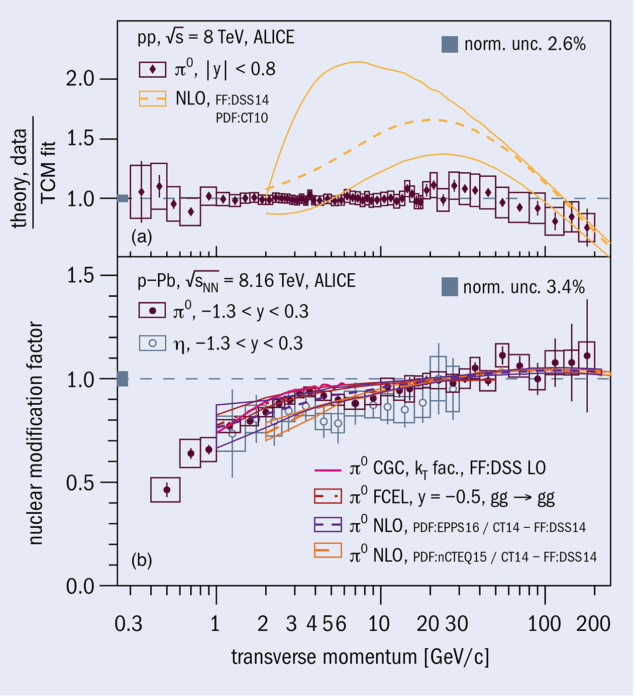# Light neutral mesons probed to high pT

30 April 2021

A report from the ALICE experimentFig. 1. (a) Neutral pion cross section compared to NLO pQCD calculations in pp collisions at 8 TeV, normalised to a phenomenological two-component model (TCM) fit of the data.(b) Nuclear modification factor of π0 and η mesons in p–Pb collisions at 8.16 TeV compared to calculations based on NLO pQCD with nuclear PDFs, the Color Glass Condensate (CGC) framework, and pQCD with final-state energy loss (FCEL). Credit: CERN

Neutral pion (π0) and eta-meson (η) production cross sections at midrapidity have recently been measured up to unprecedentedly high transverse momenta (pT) in proton–proton (pp) and proton–lead (p–Pb) collisions at √sNN = 8 and 8.16 TeV, respectively. The mesons were reconstructed in the two-photon decay channel for pT from 0.5 and 1 GeV up to 200 and 50 GeV for π0 and η mesons, respectively. The high momentum reach for the π0 measurement was achieved by identifying two-photon showers reconstructed as a single energy deposit in the ALICE electromagnetic calorimeter.

In pp collisions, measurements of identified hadron spectra are used to constrain perturbative predictions from quantum chromodynamics (QCD). At large momentum transfer (Q2), one relies in these perturbative approximations of QCD (pQCD) on the factorisation of computable short-range parton scattering processes such as quark–quark, quark–gluon and gluon–gluon scatterings from long-range properties of QCD that need experimental input. These properties are modelled by parton distribution functions (PDFs), which describe the fractional-momentum (x) distributions of quarks and gluons within the proton, and fragmentation functions, which describe the fractional-momentum distribution of quarks or gluons for hadrons of a certain species.

In p–Pb collisions, nuclear effects are expected to significantly affect particle production, in particular at small parton fractional momentum x, compared to pp collisions. Modification at low pT (~1 GeV), usually attributed to nuclear shadowing (CERN Courier March/April 2021 p19), can be parameterised by nuclear parton distribution functions (nPDFs). However, since high parton densities are reached at the LHC, the Colour Glass Condensate (CGC) framework is also applicable at low pT (x values as small as ~5 × 10–4), which predicts strong particle suppression due to saturation of the parton phase space in nuclei. Above momenta of about 10 GeV/c, measurements in p–Pb collisions can also be sensitive to the energy loss of the outgoing partons in nuclear matter.

The nuclear modification factor (RpPb), shown in the lower panel of the figure, was measured as the ratio of the cross sections in p–Pb and pp collisions normalised by the atomic mass number. Below 10 GeV, RpPb is found to be smaller than unity, while above 10 GeV it is consistent with unity. The measurement is described by calculations over the full transverse momentum range and provides further constraints to the nPDF parameterisations for lower than about 5 GeV. The direct comparison of the neutral pion cross section in pp collisions at 8 TeV, with pQCD calculations shown in the upper panel of the figure, reveals differences in the low to intermediate pT range, which, however, cancel in RpPb, since similar differences are also present for the p–Pb cross section. Future high-precision measurements are ongoing using the large dataset from pp collisions at 13 TeV, providing further constraints to pQCD calculations.